NEET  >  Test: Boyle's & Charles Law

# Test: Boyle's & Charles Law - NEET

Test Description

## 14 Questions MCQ Test Chemistry Class 11 - Test: Boyle's & Charles Law

Test: Boyle's & Charles Law for NEET 2023 is part of Chemistry Class 11 preparation. The Test: Boyle's & Charles Law questions and answers have been prepared according to the NEET exam syllabus.The Test: Boyle's & Charles Law MCQs are made for NEET 2023 Exam. Find important definitions, questions, notes, meanings, examples, exercises, MCQs and online tests for Test: Boyle's & Charles Law below.
Solutions of Test: Boyle's & Charles Law questions in English are available as part of our Chemistry Class 11 for NEET & Test: Boyle's & Charles Law solutions in Hindi for Chemistry Class 11 course. Download more important topics, notes, lectures and mock test series for NEET Exam by signing up for free. Attempt Test: Boyle's & Charles Law | 14 questions in 14 minutes | Mock test for NEET preparation | Free important questions MCQ to study Chemistry Class 11 for NEET Exam | Download free PDF with solutions
 1 Crore+ students have signed up on EduRev. Have you?
Test: Boyle's & Charles Law - Question 1

### Direction (Q. Nos. 1-4) This section contains 4 multiple choice questions. Each question has four choices (a), (b), (c) and (d), out of which ONLY ONE option is correct. Q. A sample of an ideal gas occupies 20 L under a pressure of 0.5 atm and under isothermal condition. On increasing its pressure to 1.0 atm, its volume will be

Detailed Solution for Test: Boyle's & Charles Law - Question 1

Since the system is isothermal, so temperature is constant.
According to Boyle's lawP1V1= P2V2
20×0.5=1×V2
10=V2

Test: Boyle's & Charles Law - Question 2

### A 10.0 cm column of air is trapped by a column of Hg 4.00 cm long in a capillary tube of uniform bore when the tube is held horizontally at 1 atm. Length of the air column when the tube is held vertically with the open end up is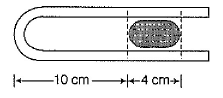Detailed Solution for Test: Boyle's & Charles Law - Question 2

V1 = 10xcm³
V2 = ?
P1 = 1atm = 760mmHg
P2 = (760 + 4)mmHg
P1V1 = P2V2
V2 = P1V1 / P2
= 760 × 10x / 764
= 9.5x
Therefore,
= 9.5x / x = 9.5

Test: Boyle's & Charles Law - Question 3

### Volume of an ideal gas is to be decreased by 10% by increase of pressure by x% under isothermal condition. Thus, x is

Detailed Solution for Test: Boyle's & Charles Law - Question 3

Applying Boyle 's law P1V1=P2V2 as temp is constant.
So let initial pressure =P initial volume =V
Now final volume V2=V-10%of V =9V/10
PV=P2×9V/10 so P2=10P/9
Therefore increment in Pressure=P/9P×100%=100/9

Test: Boyle's & Charles Law - Question 4

Density of an ideal gas at 298 K and 1.0 atm is found to be 1.25 kg m-3. Density of the gas at 1.5 atm and at 298 K is

Detailed Solution for Test: Boyle's & Charles Law - Question 4

We know ideal gas equation,
PV=nRT.
n= m(mass)/M(molecular weight).
PV=m/M RT.
PM=m/v RT.
density (d)=m(mass)/v (volume).
PM=dRT.
d=PM/RT.
here, density is directly proportional to pressure and inversely proportional to temperature...
d1/d2=P1 T2 / P2 T1.
1.25/d2= 1×298/1.5×298.
1.25/d2=1/1.5.
d2= 1.25×1.5.
d2=1.875

Test: Boyle's & Charles Law - Question 5

Direction (Q. Nos. 5-9) This section contains 5 multiple choice questions. Each question has four choices (a), (b), (c) and (d), out of which ONE or  MORE THANT ONE  is correct.

Q. An ideal gas is at 300 K and 1 atm. Then volume remains constant if

Detailed Solution for Test: Boyle's & Charles Law - Question 5

We know that
pV = nRT
Or V = nRT/p
If we increase both T and P 2 times, only then the volume remains constant.

Test: Boyle's & Charles Law - Question 6

The pressure of a 1:4 mixture of dihydrogen and dioxygen enclosed in a vessel is one atmosphere. What would be the partial pressure of dioxygen?

Detailed Solution for Test: Boyle's & Charles Law - Question 6

Let the number of moles of dihydrogen and dioxygen be 1 and 4.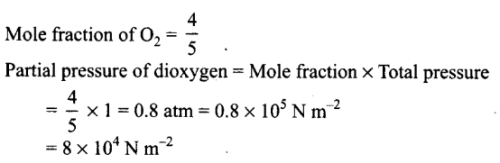*Multiple options can be correct
Test: Boyle's & Charles Law - Question 7

Select correct matching of laws with their corresponding relations.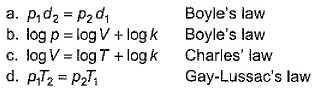Detailed Solution for Test: Boyle's & Charles Law - Question 7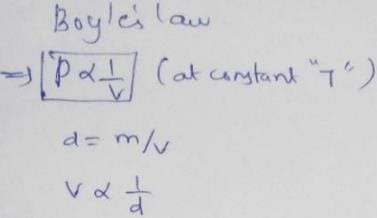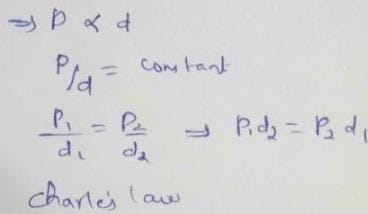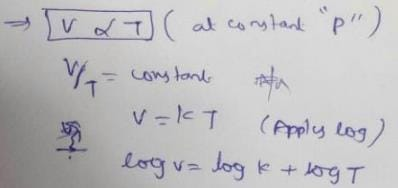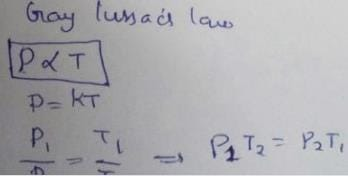Test: Boyle's & Charles Law - Question 8

Charle's law defines value of 'k' as

Detailed Solution for Test: Boyle's & Charles Law - Question 8

According to Charles law,at constant pressure, V∝T
Or V/T = k

*Multiple options can be correct
Test: Boyle's & Charles Law - Question 9

Consider gases confined by a liquid, as shown in figure.
Density of the liquid = d (all values in SI unit)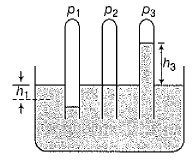Then

Detailed Solution for Test: Boyle's & Charles Law - Question 9

In this setup
The liquid in tube 1 has got lowered by height h1 which means that the pressure inside the tube is greater than the pressure exerted by the atmosphere. This excess pressure must be equal to the pressure exerted by h1 column of liquid of the same cross section ,therefore
Pressure inside tube 1=atmospheric pressure+h1dg
In tube 2 since the level of water is same as that outside the tube so her
Pressure in tube 2=atmospheric pressure
In tube 3 the liquid has risen by height be which means that pressure inside the tube is less than the pressure exerted by atmospheric pressure .
Atmospheric pressure=pressure in tube+h3dg
Pressure in tube=atmospheric pressure -h3dg

Test: Boyle's & Charles Law - Question 10

Direction (Q. Nos. 10-11) This section contains a paragraph, wach describing theory, experiments, data etc. three Questions related to paragraph have been given.Each question have only one correct answer among the four given options (a),(b),(c),(d).

For Charles’ law, graphical representation between log V and log T under isobaric condition is shown below for equation V = kT.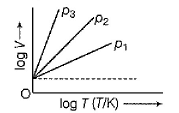Q. Relation between p1, p2 and p3 is

Detailed Solution for Test: Boyle's & Charles Law - Question 10

From  ideal gas equation, we have
pV = nRT
Or V = nRT/p
Taing log on both sides
log V = log nRT - log p
Or y = mx - c
Let us make a point constant on time axis like this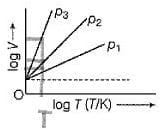Now we have the temperature constant.So to have maximum value of log V, we need to have minimum value of log p. From the graph we can see that we have least pressure for p3 . So the order will be p3<p2<p1.

Test: Boyle's & Charles Law - Question 11

For Charles’ law, graphical representation between log V and log T under isobaric condition is shown below for equation V = kTQ. Volume V = constant k, when

Detailed Solution for Test: Boyle's & Charles Law - Question 11

-272deg.
For C = 1 K
V / 1 = K
V = K

Test: Boyle's & Charles Law - Question 12

Direction (Q. Nos. 12) Choice the correct combination of elements and column I and coloumn II  are given as option (a), (b), (c) and (d), out of which ONE option is correct.

Q.
G
raphicaily Boyle’s law can be represented by various options depending on coordinates. Match the graphs in Column t with their coordinates in Column II and select the correct alternate from the codes given beiow.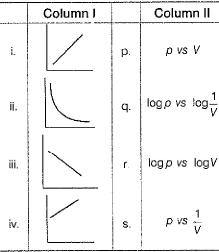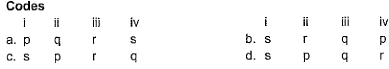Detailed Solution for Test: Boyle's & Charles Law - Question 12

From Boyle’s law;
p ∝ 1/V ⇒ Graph i (Straight line passing through origin)
Or pV = Constant ⇒ Graph ii (rectangular hyperbola)
Taking log on both side;
log(pV) = logK
logp + logV = k’
logp = -logV = k’ (y = -mx+c) ⇒ Graph iii
log p = log V-1 + k’
Logp = log(1/V) + k’ ⇒ Graph iv
So option c is correct

*Answer can only contain numeric values
Test: Boyle's & Charles Law - Question 13

Direction (Q. Nos. 13 and 14) This section contains 2 questions. when worked out will result in an integer from 0 to 9 (both inclusive).

Isothermally compressibility of an ideal gas is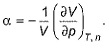Q.
What is the value of a at 0.2 bar?

Detailed Solution for Test: Boyle's & Charles Law - Question 13

α = -1/V(dV/dP)T
pV = RT;
V = RT/p
dV/dp = -RT/p2
α = -1/(RT/p)×(-RT/p2)
α = 1/p
Or α =  (since p = 0.2)

*Answer can only contain numeric values
Test: Boyle's & Charles Law - Question 14

Cubic expansion coefficient (β) for an ideal gas is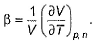Q.
Its value at 250 K is x * 10-3 K -1. What is value of x?

Detailed Solution for Test: Boyle's & Charles Law - Question 14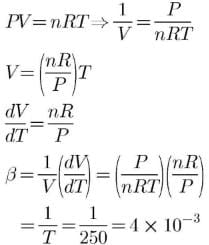## Chemistry Class 11

200 videos|339 docs|229 tests
Information about Test: Boyle's & Charles Law Page
In this test you can find the Exam questions for Test: Boyle's & Charles Law solved & explained in the simplest way possible. Besides giving Questions and answers for Test: Boyle's & Charles Law, EduRev gives you an ample number of Online tests for practice

## Chemistry Class 11

200 videos|339 docs|229 tests

### How to Prepare for NEET

Read our guide to prepare for NEET which is created by Toppers & the best Teachers(Scan QR code)